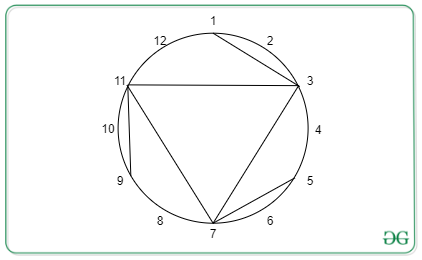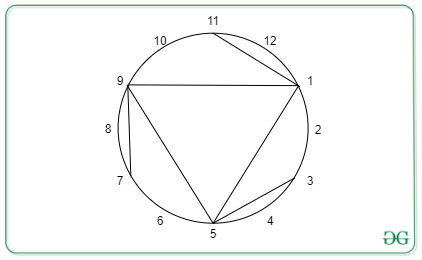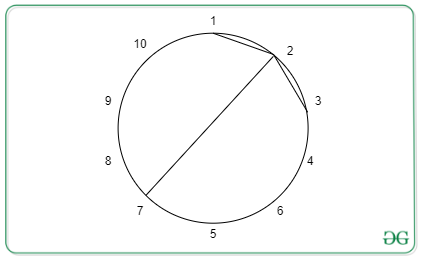Open in App
Not now

# Check if chords of a Circle are symmetric after some rotation

• Difficulty Level : Hard
• Last Updated : 08 Jun, 2022

Given two Integers N and M, N indicating equidistant points on circumference of a circle and M indicating number of chords formed with those points. Also given is a vector of pairs C containing position of chords. The task is to rotate the circle by any degree, say X, where 0 < X < 360, and check if the chords of are still symmetric to the original circle.

Example:

Input: N = 12, M = 6, C = {{1, 3}, {3, 7}, {5, 7}, {7, 11}, {9, 11}, {11, 3}};
Output: YESOriginalAfter Rotation

Input: N = 10, M = 3,  C = {{1, 2}, {3, 2}, {7, 2}}
Output: NONo rotational symmetry possible

Naive Approach: Rotate for every distance K in the range[1, N] and check for each point [a, b] if the rotated point [a + K, b + K] exits.
If there exists any k then print YES else print NO
Time Complexity: O(N*M)

Efficient Approach: It is enough to check for the divisors of N
Let us suppose if we rotate the image by K units then the whole image will be divided into N/K blocks. Then if K is not a divisor of N, there will be an asymmetric block of length less than K and the image will never be symmetric to the original figure.
So calculate all the divisors of N and check for each chord the rotated chord exists or not.

Below is the implementation of the above approach:

## C++

 `// C++ Program to for the above approach`   `#include ` `using` `namespace` `std;`   `// Utility function to calculate` `// divisors of a number in O(sqrt(N))` `vector<``int``> calculateDivisors(``int` `N)` `{` `    ``vector<``int``> ``div``;` `    ``for` `(``int` `i = 1; i * i <= N; i++) {` `        ``if` `(N % i == 0) {` `            ``div``.push_back(i);` `            ``if` `(N / i != i && i != 1) {` `                ``div``.push_back(N / i);` `            ``}` `        ``}` `    ``}` `    ``return` `div``;` `}` `int` `checkRotationallySymmetric(` `    ``vector > A,` `    ``int` `N, ``int` `M)` `{` `    ``// Maintain a set to check quickly` `    ``// the presence of a chord` `    ``set > st;` `    ``for` `(``int` `i = 0; i < M; i++) {` `        ``--A[i].first, --A[i].second;` `        ``if` `(A[i].first > A[i].second) {` `            ``swap(A[i].first, A[i].second);` `        ``}` `        ``st.insert(A[i]);` `    ``}`   `    ``// Calculate the divisors of N.` `    ``vector<``int``> ``div` `= calculateDivisors(N);`   `    ``// Iterate through the divisors` `    ``for` `(``auto` `x : ``div``) {` `        ``bool` `exist = 1;` `        ``for` `(``int` `i = 0; i < M; i++) {` `            ``int` `dx = (A[i].first + x) % N;` `            ``int` `dy = (A[i].second + x) % N;` `            ``if` `(dx > dy) {` `                ``swap(dx, dy);` `            ``}` `            ``if` `(st.find({ dx, dy }) != st.end()) {`   `                ``// There exists a valid` `                ``// chord after rotation` `            ``}` `            ``else` `{`   `                ``// There is no valid chord after rotation` `                ``exist = ``false``;` `                ``break``;` `            ``}` `        ``}`   `        ``// if there exist another chord after` `        ``// rotation for every other chord print` `        ``// YES and exit the function` `        ``if` `(exist) {` `            ``cout << ``"YES"``;` `            ``return` `0;` `        ``}` `    ``}` `    ``cout << ``"NO"``;` `    ``return` `0;` `}`   `// Driver Code` `int` `main()` `{` `    ``int` `N = 12, M = 6;` `    ``vector > C` `        ``= { { 1, 3 }, { 3, 7 }, { 5, 7 },` `             ``{ 7, 11 }, { 9, 11 }, { 11, 3 } };` `    ``checkRotationallySymmetric(C, N, M);` `    ``return` `0;` `}`

## Javascript

 `// JavaScript Program to for the above approach`   `// Utility function to calculate` `// divisors of a number in O(sqrt(N))` `function` `calculateDivisors(N)` `{` `    ``let div = ``new` `Array();` `    ``for` `(let i = 1; i * i <= N; i++) {` `        ``if` `(N % i == 0) {` `            ``div.push(i);` `            ``if` `(Math.floor(N / i) != i && i != 1) {` `                ``div.push(Math.floor(N / i));` `            ``}` `        ``}` `    ``}`   `    ``return` `div;` `}` `function` `checkRotationallySymmetric( A, N, M)` `{` `    ``// Maintain a set to check quickly` `    ``// the presence of a chord` `    ``let st = ``new` `Set();` `    ``for` `(let i = 0; i < M; i++) {` `        ``A[i] = A[i] - 1;` `        ``A[i] = A[i] - 1;` `        ``if` `(A[i] > A[i]) {` `            ``let temp = A[i];` `            ``A[i] = A[i];` `            ``A[i] = temp;` `        ``}` `        ``st.add(A[i].join(``''``));` `    ``}`   `    ``// Calculate the di   ors of N.` `    ``let div = calculateDivisors(N);` `    `  `    ``// Iterate through the divisors` `    ``for` `(let j = 0; j < div.length; j++){` `        `  `        ``let exist = ``true``;` `        ``for` `(let i = 0; i < M; i++) {` `            ``let dx = (A[i] + div[j]) % N;` `            ``let dy = (A[i] + div[j]) % N;` `            ``if` `(dx > dy) {` `                `  `                ``// swapping dx and dy. ` `                ``let temp = dx;` `                ``dx = dy;` `                ``dy = temp;` `            ``}` `            `  `            ``if` `(st.has([dx, dy].join(``''``))) {` `                ``// There exists a valid` `                ``// chord after rotation` `            ``}` `            ``else` `{` `                ``// console.log([dx, dy]);` `                ``// There is no valid chord after rotation` `                ``exist = ``false``;` `                ``break``;` `            ``}` `        ``}`   `        ``// if there exist another chord after` `        ``// rotation for every other chord print` `        ``// YES and exit the function` `        ``if` `(exist) {` `            ``console.log(``"YES"``);` `            ``return` `0;` `        ``}` `    ``}` `    ``console.log(``"NO"``);` `    ``return` `0;` `}`   `// Driver Code` `let N = 12, M = 6;` `let C` `    ``= [[1, 3 ], [3, 7 ], [5, 7 ],` `         ``[7, 11 ], [9, 11], [11, 3 ]];` `checkRotationallySymmetric(C, N, M);`   `// The code is contributed by Gautam goel (gautamgoel962)`

Output

`YES`

Time Complexity: O(M*sqrt(N)*log M)
Space Complexity: O(M)

My Personal Notes arrow_drop_up
Related Articles